### Home > AC > Chapter 8 > Lesson 8.1.2 > Problem8-16

8-16.

Use the process you developed in problem 8-13 to factor the following quadratics, if possible.

1. $x^2 − 4x − 12$

1. $4x^2 + 4x + 1$

1. $2x^2 − 9x − 5$

1. $3x^2 + 10x − 8$

Use a generic rectangle and a diamond to factor the quadratic.

(x − 6) (x + 2)

See the hints in part (a).

(2x + 1)2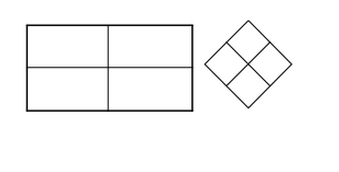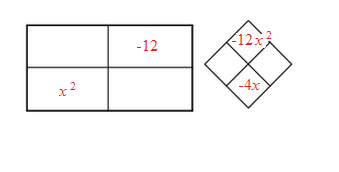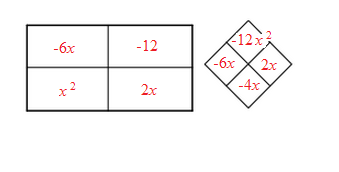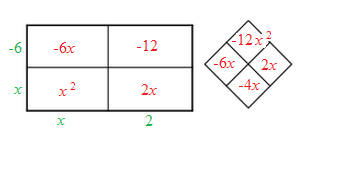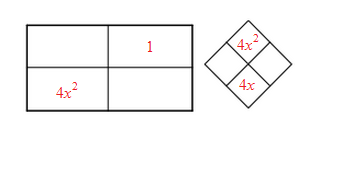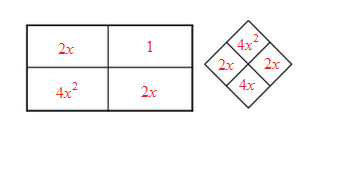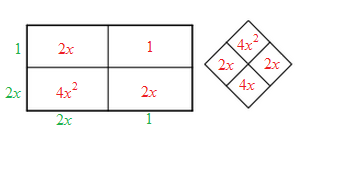See the hints in part (a).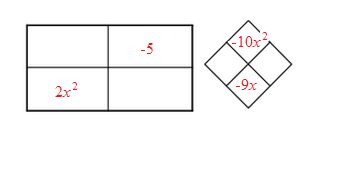See the hints in part (a).